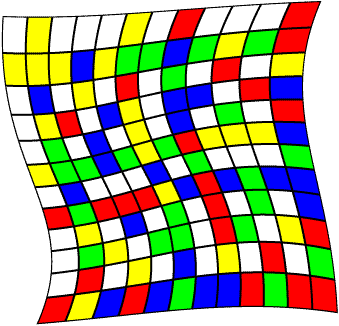Matlab Code to Draw Fibonacci CheckerboardsHere is the Matlab code that generates the Fibonacci checkerboards. The variable n specifies what modulo is used, and hence the size of the board (it will be nxn). The a and b parameters choose which recursion is used to generate the sequence. As you change n, you will want to choose different color maps so that the board looks good. Standard rules for slide checkers are here.

 % draw fibonacci checkerboards % by Bill Sethares clear n=12; a = 1; b = 1; % standard fibonacci checkerboard checks = zeros(n+1); thiscolor = 0; for i=1:n    for j=1:n      if checks(i,j)==0      thiscolor = thiscolor+1;      irun = i;      jrun = j;      checks(irun,jrun)=thiscolor;      k=1;      while kTo get to my homepage, click here.